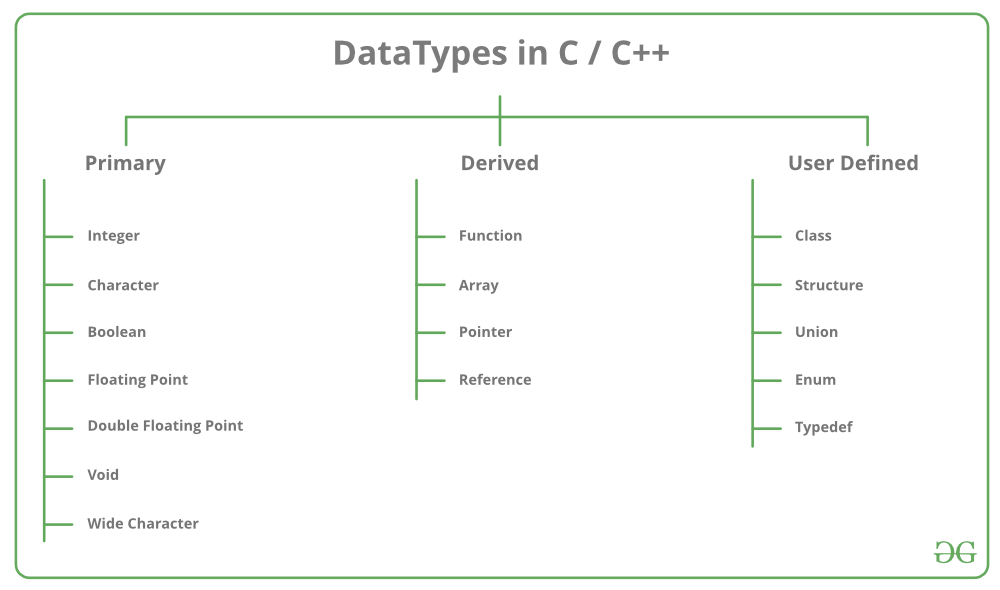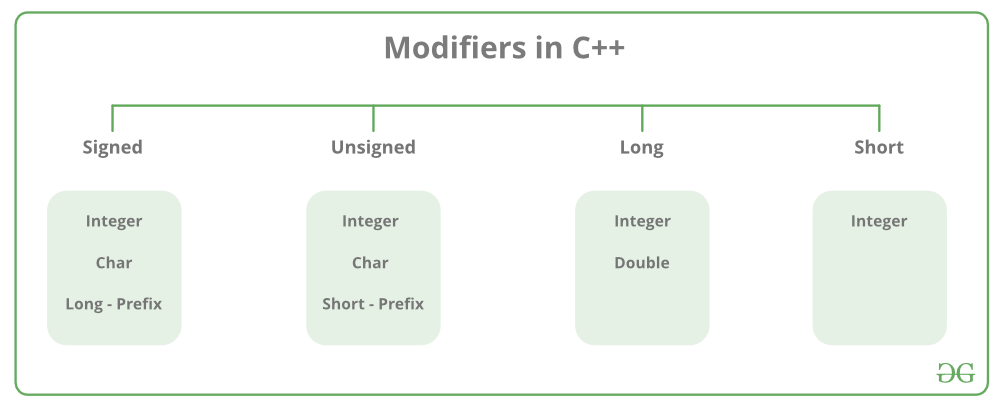# C++ Data Types

• Difficulty Level : Easy
• Last Updated : 26 May, 2022

All variables use data-type during declaration to restrict the type of data to be stored. Therefore, we can say that data types are used to tell the variables the type of data it can store. Whenever a variable is defined in C++, the compiler allocates some memory for that variable based on the data type with which it is declared. Every data type requires a different amount of memory.Data types in C++ are mainly divided into three types:

1. Primitive Data Types: These data types are built-in or predefined data types and can be used directly by the user to declare variables. example: int, char, float, bool, etc. Primitive data types available in C++ are:

• Integer
• Character
• Boolean
• Floating Point
• Double Floating Point
• Valueless or Void
• Wide Character

2. Derived Data Types: The data types that are derived from the primitive or built-in datatypes are referred to as Derived Data Types. These can be of four types namely:

• Function
• Array
• Pointer
• Reference

3. Abstract or User-Defined Data Types: These data types are defined by the user itself. Like, as defining a class in C++ or a structure. C++ provides the following user-defined datatypes:

• Class
• Structure
• Union
• Enumeration
• Typedef defined DataType

• Integer: The keyword used for integer data types is int. Integers typically require 4 bytes of memory space and range from -2147483648 to 2147483647.
• Character: Character data type is used for storing characters. The keyword used for the character data type is char. Characters typically require 1 byte of memory space and range from -128 to 127 or 0 to 255.
• Boolean: Boolean data type is used for storing boolean or logical values. A boolean variable can store either true or false. The keyword used for the boolean data type is bool
• Floating Point: Floating Point data type is used for storing single-precision floating-point values or decimal values. The keyword used for the floating-point data type is float. Float variables typically require 4 bytes of memory space.
• Double Floating Point: Double Floating Point data type is used for storing double-precision floating-point values or decimal values. The keyword used for the double floating-point data type is double. Double variables typically require 8 bytes of memory space.
• void: Void means without any value. void data type represents a valueless entity. A void data type is used for those function which does not return a value.
• Wide Character: Wide character data type is also a character data type but this data type has a size greater than the normal 8-bit datatype. Represented by wchar_t. It is generally 2 or 4 bytes long.

### Datatype Modifiers

As the name implies, datatype modifiers are used with the built-in data types to modify the length of data that a particular data type can hold.Data type modifiers available in C++ are:

• Signed
• Unsigned
• Short
• Long

The below table summarizes the modified size and range of built-in datatypes when combined with the type modifiers:

Note: Above values may vary from compiler to compiler. In the above example, we have considered GCC 32 bit.
We can display the size of all the data types by using the sizeof() operator and passing the keyword of the datatype as an argument to this function as shown below:

Now to get the range of data types refer to the following chart

Note: syntax<limits.h> header file is defined to find the range of fundamental data-types. Unsigned modifiers have minimum value is zero. So, no macro constants are defined for the unsigned minimum value.

Macro Constants

Name                                                                                                                             Expresses
CHAR_MIN                                                              Minimum value for an object of type char
CHAR_MAX                                                                Maximum value for an object of type char
SCHAR_MIN                                                             Minimum value for an object of type Signed char
SCHAR_MAX                                                                Maximum value for an object of type Signed char
UCHAR_MAX                                                                 Maximum value for an object of type Unsigned char
CHAR_BIT                                                                 Number of bits in a char object
MB_LEN_MAX                                                                 Maximum number of bytes in a multi-byte character
SHRT_MIN                                                                 Minimum value for an object of type short int
SHRT_MAX                                                                 Maximum value for an object of type short int
USHRT_MAX                                                                 Maximum value for an object of type Unsigned short int
INT_MIN                                                                 Minimum value for an object of type int
INT_MAX                                                                 Maximum value for an object of type int
UINT_MAX                                                                 Maximum value for an object of type Unsigned int
LONG_MIN                                                                 Minimum value for an object of type long int
LONG_MAX                                                                 Maximum value for an object of type long int
ULONG_MAX                                                                 Maximum value for an object of type Unsigned long int
LLONG_MIN                                                                 Minimum value for an object of type long long int
LLONG_MAX                                                                 Maximum value for an object of type long long int
ULLONG_MAX                                                                 Maximum value for an object of type Unsigned long long int

The actual value depends on the particular system and library implementation but shall reflect the limits of these types in the target platform. LLONG_MIN, LLONG_MAX, and ULLONG_MAX are defined for libraries complying with the C standard of 1999 or later (which only includes the C++ standard since 2011: C++11).

C++ Program to Find the Range of Data Types using Macro Constants

## CPP

 `// C++ program to sizes of data types``#include ``#include ``using` `namespace` `std;` `int` `main()``{``    ``cout << ``"Size of char : "` `<< ``sizeof``(``char``) << ``" byte"``         ``<< endl;``  ``cout << ``"char minimum value: "` `<< CHAR_MIN << endl;``  ` `  ``cout << ``"char maximum value: "` `<< CHAR_MAX << endl;``  ` `  ` `    ``cout << ``"Size of int : "` `<< ``sizeof``(``int``) << ``" bytes"``         ``<< endl;``    ``cout << ``"Size of short int : "` `<< ``sizeof``(``short` `int``)``         ``<< ``" bytes"` `<< endl;``    ``cout << ``"Size of long int : "` `<< ``sizeof``(``long` `int``)``         ``<< ``" bytes"` `<< endl;``    ``cout << ``"Size of signed long int : "``         ``<< ``sizeof``(``signed` `long` `int``) << ``" bytes"` `<< endl;``    ``cout << ``"Size of unsigned long int : "``         ``<< ``sizeof``(unsigned ``long` `int``) << ``" bytes"` `<< endl;``    ``cout << ``"Size of float : "` `<< ``sizeof``(``float``) << ``" bytes"``         ``<< endl;``    ``cout << ``"Size of double : "` `<< ``sizeof``(``double``)``         ``<< ``" bytes"` `<< endl;``    ``cout << ``"Size of wchar_t : "` `<< ``sizeof``(``wchar_t``)``         ``<< ``" bytes"` `<< endl;` `    ``return` `0;``}`

Output

```Size of char : 1 byte
Size of int : 4 bytes
Size of short int : 2 bytes
Size of long int : 8 bytes
Size of signed long int : 8 bytes
Size of unsigned long int : 8 bytes
Size of float : 4 bytes
Size of double : 8 bytes
Size of wchar_t : 4 bytes```